VHDL coding tips and tricks: Simple sine wave generator in VHDL

## Wednesday, March 17, 2010

### Simple sine wave generator in VHDL

Here is a sine wave generator in VHDL.This module outputs integer values of the wave from a look up table.In the module I have declared an array of size 30 byte ,which stores the value of sine component at different angles.If  you want to include more number of values,to increase the accuracy then you can do it in MATLAB.Type any one of the following comment in MATLAB:
t=0 : pi/10 : 2*pi ;   % for 20 values.
t=0 : pi/50 : 2*pi ;   % for 100 values etc..
(then type one of the following comment)
int32(sin(t)*10000/128)   %128 for 8 bit output.
int32(sin(t)*10000/512)   %512 for 6 bit output etc...
Now copy the values generated and paste them in the VHDL code at the appropriate place.
The more number values we use and the more bits we use,higher the accuracy is.But if the memory resources is limited then you should reduce the number of values.The code can be modified for efficient use of memory so that,only the first (pi/2) values are stored in the ROM.
If the frequency of your wave is very less then it is better you increase the number of values.Otherwise there will be lot of high frequency components in your output wave.

library IEEE;
use IEEE.STD_LOGIC_1164.ALL;
use IEEE.NUMERIC_STD.ALL;  --try to use this library as much as possible.

entity sinewave is
port (clk :in  std_logic;
dataout : out integer range -128 to 127
);
end sinewave;

architecture Behavioral of sinewave is
signal i : integer range 0 to 30:=0;
type memory_type is array (0 to 29) of integer range -128 to 127;
--ROM for storing the sine values generated by MATLAB.
signal sine : memory_type :=(0,16,31,45,58,67,74,77,77,74,67,58,45,31,16,0,
-16,-31,-45,-58,-67,-74,-77,-77,-74,-67,-58,-45,-31,-16);

begin

process(clk)
begin
--to check the rising edge of the clock signal
if(rising_edge(clk)) then
dataout <= sine(i);
i <= i+ 1;
if(i = 29) then
i <= 0;
end if;
end if;
end process;

end Behavioral;
Let me make some comments about the above code:
1) I have used  the library "NUMERIC_STD" instead of "STD_LOGIC_ARITH." and "STD_LOGIC_UNSIGNED" here.The reason is that the last two libraries are not approved by IEEE.The standard IEEE library is "numeric_std".So try to use this library as much as possible.Also using std_logic_vector every where is not a good method of coding.Use integer or signed instead.In the old codes I have written here, I have used these libraries,but I recommend not to use them.
2)Also, use  "rising_edge(clk)" instead of "if(clk'event and clk='1')".
3)In the same way any wave generator can be written.Only thing you have to change is the values stored in ROM.
4)The code is synthesizable.

#### 36 comments:

1.hi!

How do you do to change the frequency of your sine wave?

Jocelyn

2.@Jocelyn : The freq of the sine wave is determined by the freq of clk in the above program.Here we need 30 clk cycles for sending one full cycle of sine values.So the period of sine wave is (freq of clk/30).
Hope I am clear.

1.can u give us a tutorial on using delta-sigma DAC ?

3.heloo jocelyn!

in the above code i have simulated and runed for 30 sin values but the out put wave does not looks like sin! is their any settings reqired for getting out put wave in the form of sin as the below!

file://localhost/C:/Documents%20and%20Settings/Administrator/Desktop/enp%20final/Synthesisable%20Sine%20Wave%20Generator_files/wave.gif

4.@prahlad : I never told that the wave will look like a sine wave in the simulator.If you want to test out use a DAC,interface it with FPGA board and connect the output of DAC to an oscilloscope.

Or another thing you can do is take a graph sheet draw the output values Vs time.You will get a sine signal.If you still not getting contact me.

1.dude iam using fpga spartan 3e ... i need 2 generate sine wave ,,,,should i use dac and thn fpga to burn code

2.hi vipin can you help me in implementing PWM and random PWM in fpga...

5.really, people probably should be using the coregen dds compiler. but overall, this shows the basic concept.

A DDS will generally exploit the symmetry in the sine wave -- only 1/4th of the sine wave values are needed.

this allows a larger table to be used. using a lot of entries allows the user to increment by N, to generate other frequencies.

6.@Chris : I do agree. But if you just use core gen IP's for each and everything then how will you learn.This is a basic code where you can see how it works.I have mentioned in this post itself that,"The code can be modified for efficient use of memory so that,only the first (pi/2) values are stored in the ROM".
I just left it as an exercise for readers.

7.Thanks,
I need to learn how to use the DAC so I can play with this!!

8.how to interface the dac with the fpga ?

9.The values were generated using matlab??? Is thr a formula for d generation of sine values??

10.hi ,

i just wanna ask , if i syenthesized this code and , interface it with VGA port , would it display the sinewave figure on monitor ?

1.Absolutely not...You would need to create double buffering and index the buffers and map the pixel data to the RGB signals and sync to the pixel clock...This requires synchronization with the hsync and vsync signals...It is not a simple thing to do and I suggest you hit the books really hard if you want to learn about very advanced HDL controllers...Just because it can be done, it does not mean it is easy to do so...

11.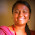can we get sine wave in modelsim

1.See my reply below...

12.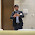how can we get sine wave in modelsim?

13.Yes, you can get a sine wave to show up in modelsim...Just view the data as signal! :)

14.Yeah, I think somebody mentioned above that this could have been done much better in four states using a quarter wave LUT and four simple states marking each quadrant...This translates 0-90 degrees would have been decimated into a LUT to whatever radians division is desired. Then on the quadrant 90-180 you would read the LUT in reverse going backwards 90-0 by reversing the indexes of the LUT and so on into the other quadrants, but at that point from 180 to 270 and 270 to 360, you'd want to negate the LUT values. That is how a quarter wave LUT works...

15.Hi there! I have generated sine wave.. But after some clock cycle the wave is not accurate..some samples are missing..I have tried adjusting the frequency but still its distorting.. For example., in the case of FSK the waves are distorting after some clock cycles.. What should I do??

16.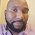Hi Vipin am working on a similar project but am not using Matlab am only using Quartus Software, how can I generate and store the sine wave values using the Quartus software

17.The calculation of the sin values is wrong. At 8 bits I would expect the maximum value to be close to 127, however this example only produces 77 as the maximum.
The correct calculation would be int32(sin(t) * 127) for 8 bit or more general int32(sin(t) * 2^(n-1)) for n bits.

Also most people don't have MATLAB available so here is some python code:
n=30;bits=8; from math import sin, pi;print([int(round(sin(x*2*pi/n)*(2**(bits-1)-1))) for x in range(n)])
"n" is the number of values and "bits" the number of bits per value.
You can try it online at https://repl.it/BcM6/1

18.can i generate sinewave using cpld?can i store look up table values in cpld and call it one by one? need help..

19.can i generate sinewave using cpld? can i store look up values in cpld and can just call it? need help

20.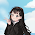How to test the code?/ what to write in the testbench

1.you dont need to enter "anything". You just run it. The only stimulation that you need is the clk. Just declare your clk period, your internal clk signal and instantiate the UUT.

21.after writing vhdl code in quartus-ii how to view the output iesinewave in modelsim..?

22.The code runs perfectly fine ie generates sine wave(i am using vivado software for running the code which allows analog sine wave output.)
But my question is that whether this sine wave could be used in other programs such as generation of ASK ,PSK by changing its phase and frequency. In other words what will be the code to change its phase and frequerncy?

23.Does vhdl support complex numbers?

24.Can we use floating point numbers in vhdl?

25.library IEEE;
use IEEE.STD_LOGIC_1164.ALL;
use IEEE.NUMERIC_STD.ALL;
use IEEE.std_logic_signed.all;

-- Uncomment the following library declaration if using
-- arithmetic functions with Signed or Unsigned values
--use IEEE.NUMERIC_STD.ALL;

-- Uncomment the following library declaration if instantiating
-- any Xilinx leaf cells in this code.
--library UNISIM;
--use UNISIM.VComponents.all;

entity lms2 is
Port (wr9,wi9,xr,xi : in STD_LOGIC_VECTOR (12 downto 0);
--u : in integer;

yr,yi,er,ei,wr8,wi8,zzz,sum1 : out STD_LOGIC_VECTOR (12 downto 0));
end lms2;

architecture Behavioral of lms2 is
signal a,b :real;
--signal yr11, yi11 : real;

--variable r,s : real range 0.0 to 15.0;

begin

process(wr9,wi9,xr,xi)--process also runs without the parameters,but it gives problems during simulation
--process(u,xr,xi)
variable wr : std_logic_vector(12 downto 0);
variable wi : std_logic_vector(12 downto 0);

--variable wr : std_logic_vector(4 downto 0) :="00010";
--variable wi : std_logic_vector(4 downto 0) :="00011";
--variable wr : std_logic_vector(4 downto 0) :=wr9;
--variable wi : std_logic_vector(4 downto 0) :=wi9;
variable xr1 : real :=3.2;
variable xi1 : real :=4.1;
variable dr1 : real :=5.2;
variable di1 : real :=4.4;
variable yr1 : real;
variable yi1 : real;
variable er1 : real;
variable ei1 : real;
variable wr1 : real := 1.8;
variable wi1 : real := 2.1;
variable u : real :=0.2;

variable f : real := 3.2;
variable g : real := 2.6;
variable h : real;
variable hh : real;

begin

h := f+g;
hh := f*g;
a <= h;
b <=hh;

--process(xr,xi,u)
--bi <= to_integer(unsigned(k)) ;
--bj <= to_integer(unsigned(l)) ;
--bk <= bi*bj;

for z in 0 to 3 loop

yr1 := wr1*xr1-wi1*xi1;--line1
yi1 := wr1*xi1+wi1*xr1;--line2

er1 := (wr1*xr1)-(wi1*xi1)-dr1;--line3
ei1 := (wr1*xi1)+(wi1*xr1)-di1;--line4

wr1 := u*(xr1*er1-xi1*ei1)+wr1;--line5
wi1 := u*(xr1*ei1+xi1*er1)+wi1;--line6

end loop;

--yr11 <= yr1;
--yi11 <= yi1;

end process;

end Behavioral;

We are implementing LMS algorithm . If we are adding line 5 and line 6 (as indicated by comments), we are getting error right from line 1 (as indicated by comments) that "non constant real valued expression not supported". However when we are omitting line 5 and line 6, we are not getting the error. Please ignore the STD_LOGIC_VECTORS(in and out)added in the beginning, as they are reserved for future use

26.how to make it suitable for a lvds dac?

27.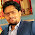what is the frequency of above sine wave??

28.What is the frequency of above sign wave and how do you modify it?

29.We are implementing LMS algorithm .

30.how simulated analog signal using ise